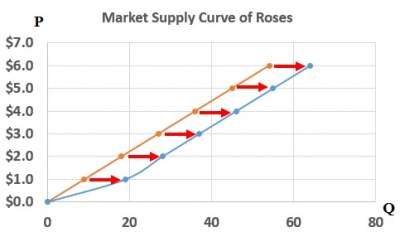# Supply Curve Shifters

### Introduction

• The supply curve shows how price has an impact on quantity supplied, other things being equal.
• "other things" are considered as non-price determinants of supply.
• Changes in them make the S curve shift.

### Supply Curve Shifters: Input Prices

• Examples of input prices such as prices of raw materials and wages.
• A decrease of input prices makes production more profitable at each output price,
hence, firms supply a larger quantity at each price, and the S curve shifts to the right.
Suppose the price of water falls. So, at each price, the quantity of roses supplied will increase for example by 10.### Supply Curve Shifters: Technology

• Technology can help to determine the quantity of inputs needed to produce a unit of output.
• Hence, a cost-saving technological enhancement has the same effect as a fall in input prices, which will shift the S curve to the right.

### Supply Curve Shifters: Number of Sellers

An increase in the number of sellers increases the quantity supplied at each price, and therefore creates a shift to the right on the S curve.

### Supply Curve Shifters: Expectations

#### Example

• A high probability of a war in the middle east leads to expectations to higher oil prices.
• Subsequently, owners of oilfields in North America and elsewhere reduce supply, save some inventory to sell later at higher price.
• This event makes the S curve to shift left.

### Exercise

Exercise on Supply Curve